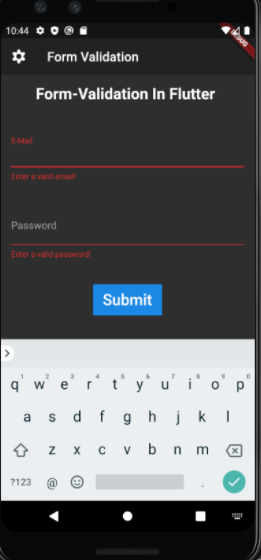GeeksforGeeks App
Open AppBrowser
Continue

# Form Validation in Flutter

Form Validation is an important part of every application. In the flutter application, there are many ways to validate form such as using a TextEditingController. But handling text controller for every Input can be messy in big applications. Hence, Form provides us a convenient way to validate user Inputs.

In form, the input is validated in your submit function(the function which is called once the user has entered every detail, But the condition is applied in each TextFormField itself with a widget name validator as shown in the below given example.

The Validator widget takes a function with a parameter that contains the value of the single input and then it checks the condition which is given in the validator function. The key is used in this because there can be many inputs so to connect the widget tree with elementary tree key of type FormState is used.

In the Code below if the input validation in the form Fails, it would lead to the following:Example:

## Dart

 `import ``'package:flutter/material.dart'``;`` ` `void` `main() => runApp(MyApp());`` ` `class` `MyApp extends StatelessWidget {``  ``@override``  ``Widget build(BuildContext context) {``    ``return` `MaterialApp(``      ``home: HomePage(),``      ``theme: ThemeData(``        ``brightness: Brightness.dark,``      ``),``    ``);``  ``}``}`` ` `class` `HomePage extends StatefulWidget {``  ``@override``  ``_HomePageState createState() => _HomePageState();``}`` ` `class` `_HomePageState extends State {``  ``var _formKey = GlobalKey();``  ``var isLoading = ``false``;`` ` `  ``void` `_submit() {``    ``final isValid = _formKey.currentState.validate();``    ``if` `(!isValid) {``      ``return``;``    ``}``    ``_formKey.currentState.save();``  ``}`` ` `  ``@override``  ``Widget build(BuildContext context) {``    ``return` `Scaffold(``      ``appBar: AppBar(``        ``title: Text(``"Form Validation"``),``        ``leading: Icon(Icons.filter_vintage),``      ``),``      ``//body``      ``body: Padding(``        ``padding: ``const` `EdgeInsets.all(16.0),``        ``//form``        ``child: Form(``          ``key: _formKey,``          ``child: Column(``            ``children: [``              ``Text(``                ``"Form-Validation In Flutter "``,``                ``style: TextStyle(fontSize: 24.0, fontWeight: FontWeight.bold),``              ``),``              ``//styling``              ``SizedBox(``                ``height: MediaQuery.of(context).size.width * 0.1,``              ``),``              ``TextFormField(``                ``decoration: InputDecoration(labelText: ``'E-Mail'``),``                ``keyboardType: TextInputType.emailAddress,``                ``onFieldSubmitted: (value) {``                  ``//Validator``                ``},``                ``validator: (value) {``                  ``if` `(value.isEmpty ||``                      ``!RegExp(r``"^[a-zA-Z0-9.a-zA-Z0-9.!#\$%&'*+-/=?^_`{|}~]+@[a-zA-Z0-9]+\.[a-zA-Z]+"``)``                          ``.hasMatch(value)) {``                    ``return` `'Enter a valid email!'``;``                  ``}``                  ``return` `null;``                ``},``              ``),``              ``//box styling``              ``SizedBox(``                ``height: MediaQuery.of(context).size.width * 0.1,``              ``),``              ``//text input ``              ``TextFormField(``                ``decoration: InputDecoration(labelText: ``'Password'``),``                ``keyboardType: TextInputType.emailAddress,``                ``onFieldSubmitted: (value) {},``                ``obscureText: ``true``,``                ``validator: (value) {``                  ``if` `(value.isEmpty) {``                    ``return` `'Enter a valid password!'``;``                  ``}``                  ``return` `null;``                ``},``              ``),``              ``SizedBox(``                ``height: MediaQuery.of(context).size.width * 0.1,``              ``),``              ``RaisedButton(``                ``padding: EdgeInsets.symmetric(``                  ``vertical: 10.0,``                  ``horizontal: 15.0,``                ``),``                ``child: Text(``                  ``"Submit"``,``                  ``style: TextStyle(``                    ``fontSize: 24.0,``                  ``),``                ``),``                ``onPressed: () => _submit(),``              ``)``            ``],``          ``),``        ``),``      ``),``    ``);``  ``}``}`

Output:

My Personal Notes arrow_drop_up